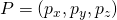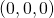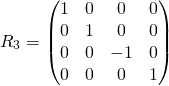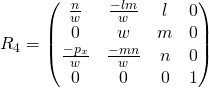# Reflection at a plane in 3D

A flat mirror in 3D is descibed by the direction cosinesof a surface normal and a point P on it’s surface.

We construct the image A’ of a point A by these steps

• translate the origin of the coordinate System to the point P
• rotate the coordingate system so, that the z-axis of the coordinate system coincides with the surface normal in P)
• mirror point A in this new coordinate system
• unrotate the coordinate system to it’s old rotation
• untranslate the origin to it’s old position

## Translation of the coordinate system in 3D so that the Origin is in P.whereare the cartesian coordinates of point P=()

Proof thatis indeed mapped to:## Rotation in 3D around the Origin (now in P) so that the z-axis coincides with the surface normalwithProof, that each pointis mapped to## Reflection at the z-axis in 3D## De-Rotation in 3Dwith## Translation in 3D back to original Position# Reflection at a plane in 3D

*** QuickLaTeX cannot compile formula:
R = R_5 R_4 R_3 R_2 R_1
<span class="ql-right-eqno">   </span><span class="ql-left-eqno">   </span><img src="https://www.optowiki.info/wp-content/ql-cache/quicklatex.com-fd64c24ef2bdf7310c7a75a3f6537980_l3.png" height="88" width="419" class="ql-img-displayed-equation quicklatex-auto-format" alt="$= \begin{pmatrix} 1-2l^2 & -2lm & -2ln & 2l(lp_x + mg + nh) \\ -2lm & 1-2m^2 & -2mn & 2m(lpx + mg + nh) \\ -2ln & -2 m n & 1-2n^2 & 2n(lpx + mg + nh) \\ 0 & 0 & 0 & 1 \end{pmatrix}$" title="Rendered by QuickLaTeX.com"/>
<span class="ql-right-eqno">   </span><span class="ql-left-eqno">   </span><img src="https://www.optowiki.info/wp-content/ql-cache/quicklatex.com-b17224e260f969ba19e366728afd8719_l3.png" height="88" width="301" class="ql-img-displayed-equation quicklatex-auto-format" alt="$= \begin{pmatrix} 1-2l^2 & -2lm & -2ln & 2lp \\ -2lm & 1-2m^2 & -2mn & 2mp \\ -2ln & -2 m n & 1-2n^2 & 2np \\ 0 & 0 & 0 & 1 \end{pmatrix}$" title="Rendered by QuickLaTeX.com"/>
Where

*** Error message:
Missing $inserted. leading text: R = R_  p = (lp_x + mp_y + np_z) =$ length from the origin to point P